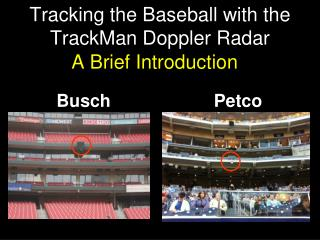# Tracking the Baseball with the TrackMan Doppler Radar - PowerPoint PPT PresentationDownload PresentationTracking the Baseball with the TrackMan Doppler Radar

Tracking the Baseball with the TrackMan Doppler RadarDownload Presentation## Tracking the Baseball with the TrackMan Doppler Radar

- - - - - - - - - - - - - - - - - - - - - - - - - - - E N D - - - - - - - - - - - - - - - - - - - - - - - - - - -
##### Presentation Transcript

1. Tracking the Baseball with the TrackMan Doppler Radar Busch Petco A Brief Introduction

2. The Doppler Technique Doppler Frequency Shift fD = Doppler Shift = FTX - FRX = 2FTX(VR/c) VR= dr/dt = rate of change of distance of ball from TM

3. Batted ball Bat Bounce Pitched ball Frequency/Velocity vs. Time Doppler shift Radial velocity Time 

4. Slightly More Detail • Want to determine location of baseball as function of time • TM determines r,, • r = distance from TM • ,=orientation angles wrt TM

5. Summary of TM Technique: r,, • Determining r(t) • Doppler shift determines dr/dt • Two-frequency phase shift determines r(0)

6. Determining r(0) • Transmit two wavelengths 1 and 2 • Initially in phase • After reflection and detection, out of phase by 2r(1/ 1 - 1/ 2) • Measuring phase shift at t=0 determines r(0)

7. Measuring angles Phase Shift Between Detectors Angles Extra distance = Dsin()  phase shift

8. Three Detectors  ,

9. Summary of Technique • Doppler shift determines dr/dt • Two wavelength phase shift determines r(0) • r(0) + dr/dt  r(t) • 3-detector array phase shifts determine (t), (t) • r(t), (t), (t) gives full 3D trajectory • Need to convert to field coordinates using location and orientation of TM

10. Measurement Principle: Spin Doppler frequency modulated by rotation frequency  Sidebands separated by n

11. TrackMan Capabilities I:Full pitched ball trajectory • Everything PITCHf/x gives plus…. • Actual release point (“extension”)  perceived velocity • Total spin (including “gyro” component) • Many more points on the trajectory Utility of more points under study

12. TrackMan Capabilities II:Full batted ball trajectory • Batted ball speed, launch & spray angles Equivalent to HITf/x • Landing point coordinates at ground level and hang time Equivalent to Hittracker • Initial spin • and more, if you want it